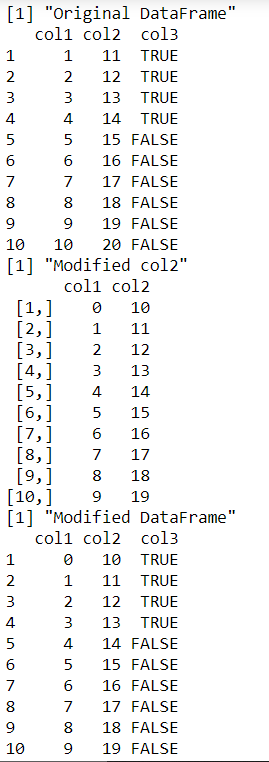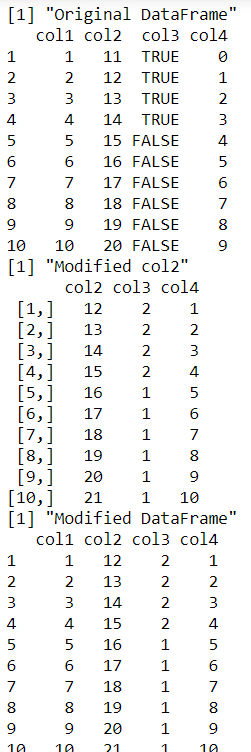Open in App
Not now

# Use apply Function Only for Specific DataFrame Columns in R

• Last Updated : 29 Sep, 2021

In this article, we are going to apply functions only for specific dataframe columns in the R language.

The function in R can be specified using the function keyword, which takes the element x, for instance, as input and modifies each of the elements in x according to the user-defined function.

```fun <- function(x){
}```

The pre-defined or user-defined function can then be applied to the specific columns of the data frame by using the inbuilt apply method in R. The apply method in R is used to apply a given function to the elements of the data frame across the specified axes. The elements are then modified. However, the factor columns must be dealt with precaution since it may lead to data loss or ambiguity. The output returned is in the form of a data frame.

Syntax: apply(data_frame[:col_indx],axes , FUN)

Arguments :

• data_frame – The data frame to apply function 2
• axes – The axes over which to apply function, 1 specifies rows and 2 columns
• FUN – The function to be applied

## R

 `# creating a data frame ` `data_frame <- ``data.frame``(col1 = ``c``(1:10),` `                         ``col2 = 11:20,` `                         ``col3 = ``c``(``rep``(``TRUE``,4),``rep``(``FALSE``,6)))` `print``(``"Original DataFrame"``)` `print``(data_frame)`   `# defining the function` `user_defined_func <- ``function``(x) {   ` `  ``# subtracting the value of 1 from each ` `  ``x-1` `}`   `data_frame_temp <- ``apply``(data_frame[ ,``c``(1,2)], 2, user_defined_func)                        ` `print``(``"Modified col2"``)` `print ``(data_frame_temp)`   `# retrieving the entire data frame` `data_frame_mod <- data_frame `   `# getting column names` `colnames <- ``colnames``(data_frame_mod)` `data_frame_mod[ , colnames %``in``% ``colnames``(data_frame_temp)] <- data_frame_temp  ` `print``(``"Modified DataFrame"``)` `print``(data_frame_mod)`

Output:### Example 2: Apply Function Only for Specific on multiple Data Frame Columns in R

The function can be applied over multiple columns in such a way that a range of columns forming a subset of the entire set of columns of the data frame is taken into account. The following code snippet illustrates the procedure where the integer value 1 is added to the last three data frame columns :

## R

 `# creating a data frame ` `data_frame <- ``data.frame``(col1 = ``c``(1:10),` `                         ``col2 = 11:20,` `                         ``col3 = ``c``(``rep``(``TRUE``,4),``rep``(``FALSE``,6)),` `                         ``col4 = 0:9)` `print``(``"Original DataFrame"``)` `print``(data_frame)`   `# defining the function` `user_defined_func <- ``function``(x) {   ` ` ``x+1` `  `  `}`   `data_frame_temp <- ``apply``(data_frame[ ,2:4],2, user_defined_func)                        ` `print``(``"Modified col2"``)` `print ``(data_frame_temp)`   `# retrieving the entire data frame` `data_frame_mod <- data_frame `   `# getting column names` `colnames <- ``colnames``(data_frame_mod)` `data_frame_mod[ , colnames %``in``% ``colnames``(data_frame_temp)] <- data_frame_temp  ` `print``(``"Modified DataFrame"``)` `print``(data_frame_mod)`

Output:Explanation: However, we notice, that since 1 is an integer value when we add its value to the logical values TRUE and FALSE of column 2 values, it leads to the ambiguous conversion of values into integers, where TRUE is taken as 1 and FALSE is mapped to an integer value of 0. The result is returned accordingly.

My Personal Notes arrow_drop_up
Related Articles